# How to Calculate and Solve for Plastic Pressure | Polymer & Textile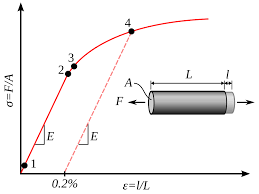The image above represents plastic pressure.

To compute for plastic pressure, two essential parameters are needed and these parameters are hydraulic pressure (PH) and intensification ratio (Ir).

The formula for calculating plastic pressure:

Pp = PH x Ir

Where;

Pp = Plastic Pressure
PH = Hydraulic Pressure
Ir = Intensification Ratio

Let’s solve an example;
Find the plastic pressure when the hydraulic pressure is 20 and the intensification ratio is 44.

This implies that;

PH = Hydraulic Pressure = 20
Ir = Intensification Ratio = 44

Pp = PH x Ir
Pp = 20 x 44
Pp = 880

Therefore, the plastic pressure is 880.

Calculating the Hydraulic Pressure when the Plastic Pressure and the Intensification Ratio is Given.

PH = Pp / Ir

Where;

PH = Hydraulic Pressure
Pp = Plastic Pressure
Ir = Intensification Ratio

Let’s solve an example;
Find the hydraulic pressure when the plastic pressure is 24 and the intensification ratio is 10.

This implies that;

Pp = Plastic Pressure = 24
Ir = Intensification Ratio = 10

PH = Pp / Ir
PH = 24 / 10
PH = 2.4

Therefore, the hydraulic pressure is 2.4.

Calculating the Intensification Ratio when the Plastic Pressure and the Hydraulic Pressure is Given.

Ir = Pp / PH

Where;

Ir = Intensification Ratio
Pp = Plastic Pressure
PH = Hydraulic Pressure

Let’s solve an example;
Find the intensification ratio when the plastic pressure is 30 and the hydraulic pressure is 15.

This implies that;

Pp = Plastic Pressure = 30
PH = Hydraulic Pressure = 15

Ir = Pp / PH
Ir = 30 / 15
Ir = 2

Therefore, the intensification ratio is 2.

Nickzom Calculator – The Calculator Encyclopedia is capable of calculating the plastic pressure.

To get the answer and workings of the plastic pressure using the Nickzom Calculator – The Calculator Encyclopedia. First, you need to obtain the app.

You can get this app via any of these means:

To get access to the professional version via web, you need to register and subscribe for NGN 1,500 per annum to have utter access to all functionalities.
You can also try the demo version via https://www.nickzom.org/calculator

Apple (Paid) – https://itunes.apple.com/us/app/nickzom-calculator/id1331162702?mt=8
Once, you have obtained the calculator encyclopedia app, proceed to the Calculator Map, then click on Polymer & Textile under Engineering.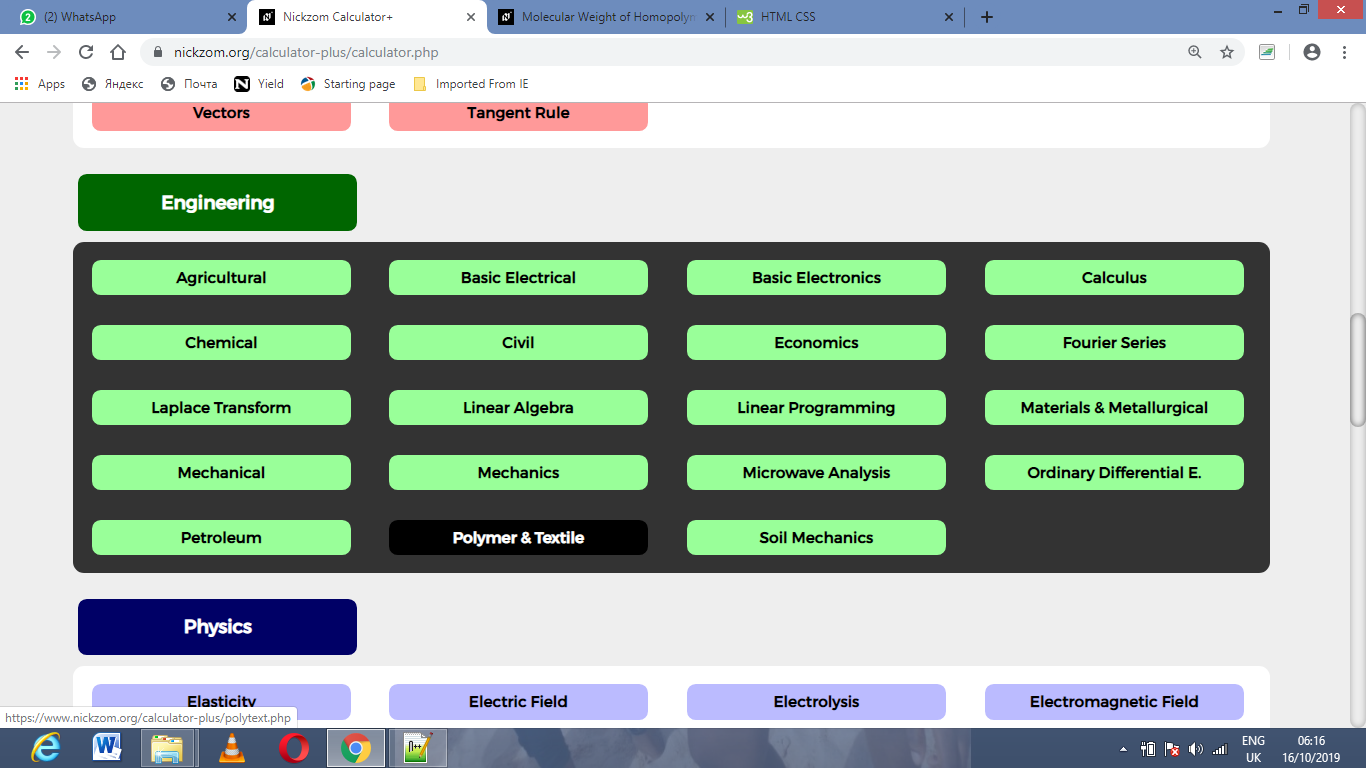Now, Click on Plastic Pressure under Polymer & Textile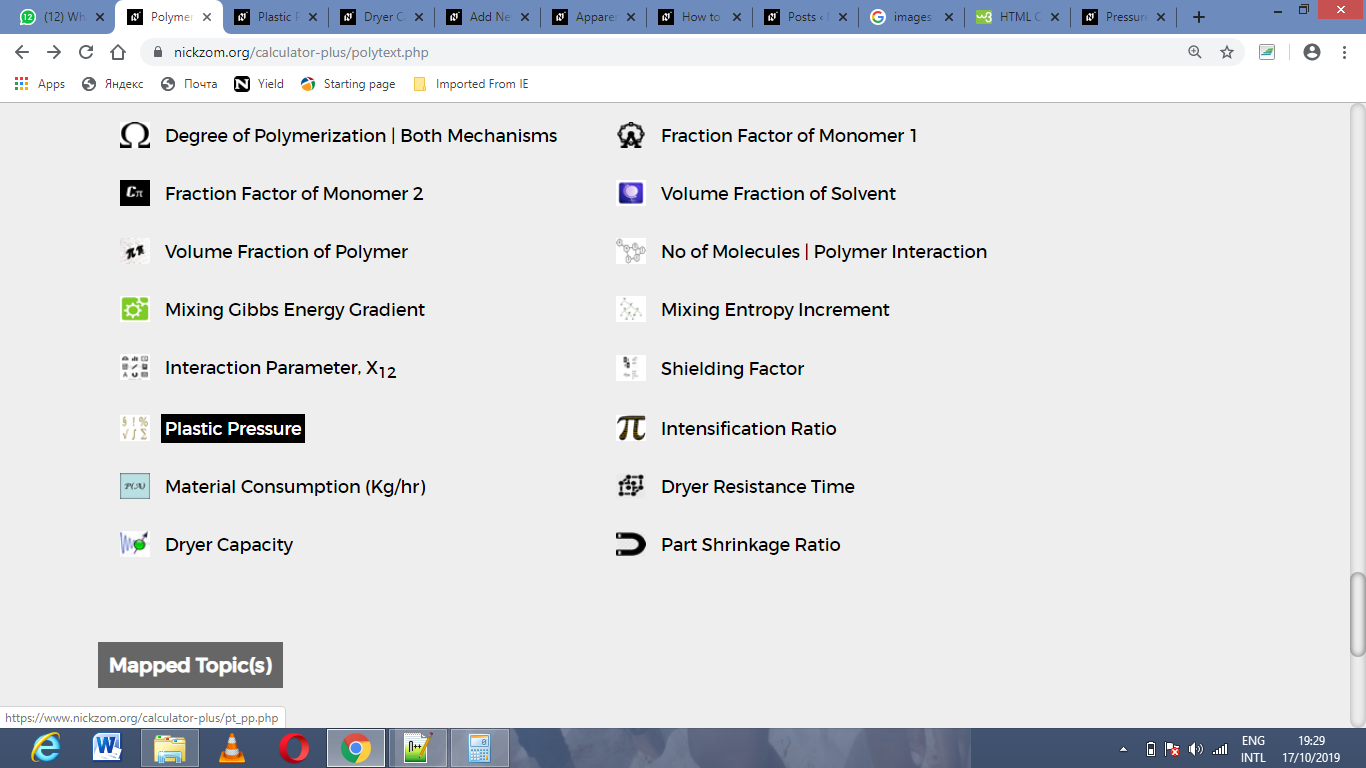The screenshot below displays the page or activity to enter your values, to get the answer for the plastic pressure according to the respective parameters which is the hydraulic pressure (PH) and intensification ratio (Ir).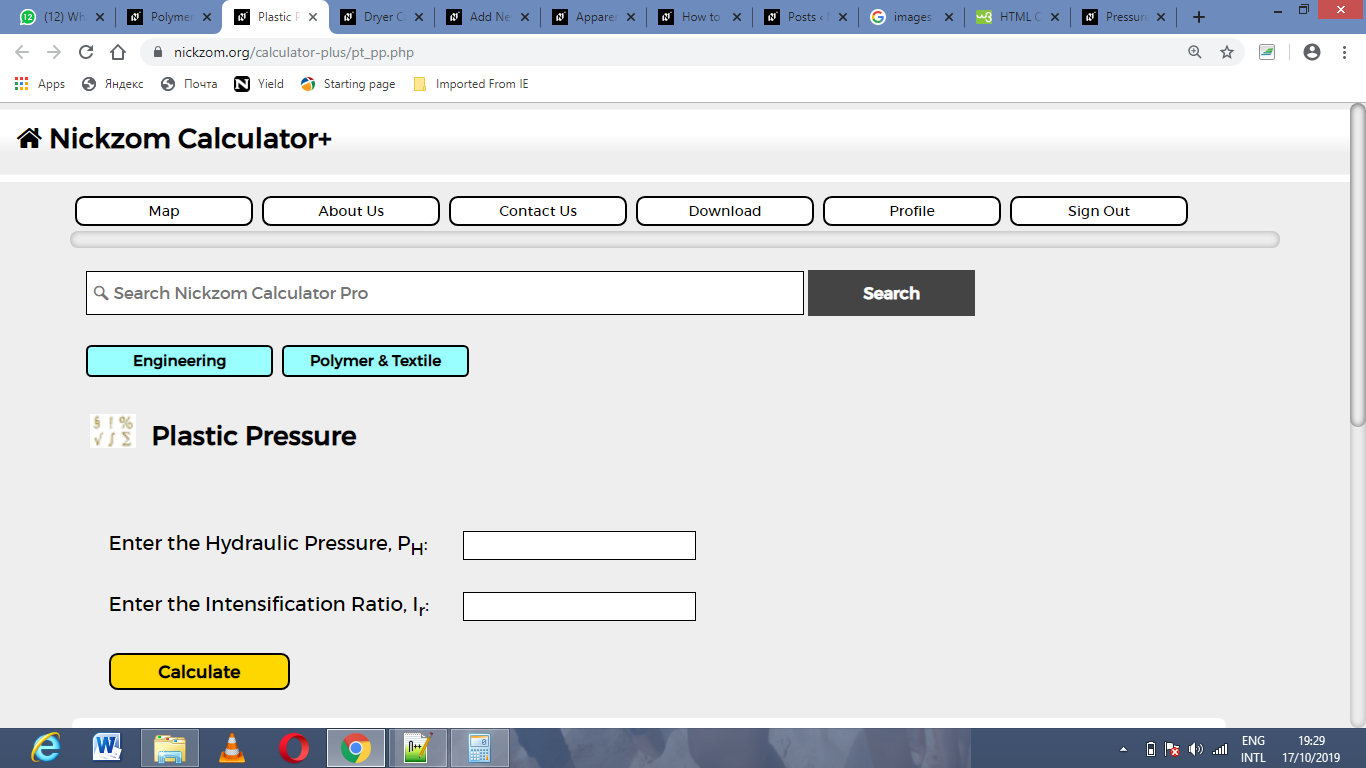Now, enter the values appropriately and accordingly for the parameters as required by the hydraulic pressure (PH) is 20 and intensification ratio (Ir) is 44.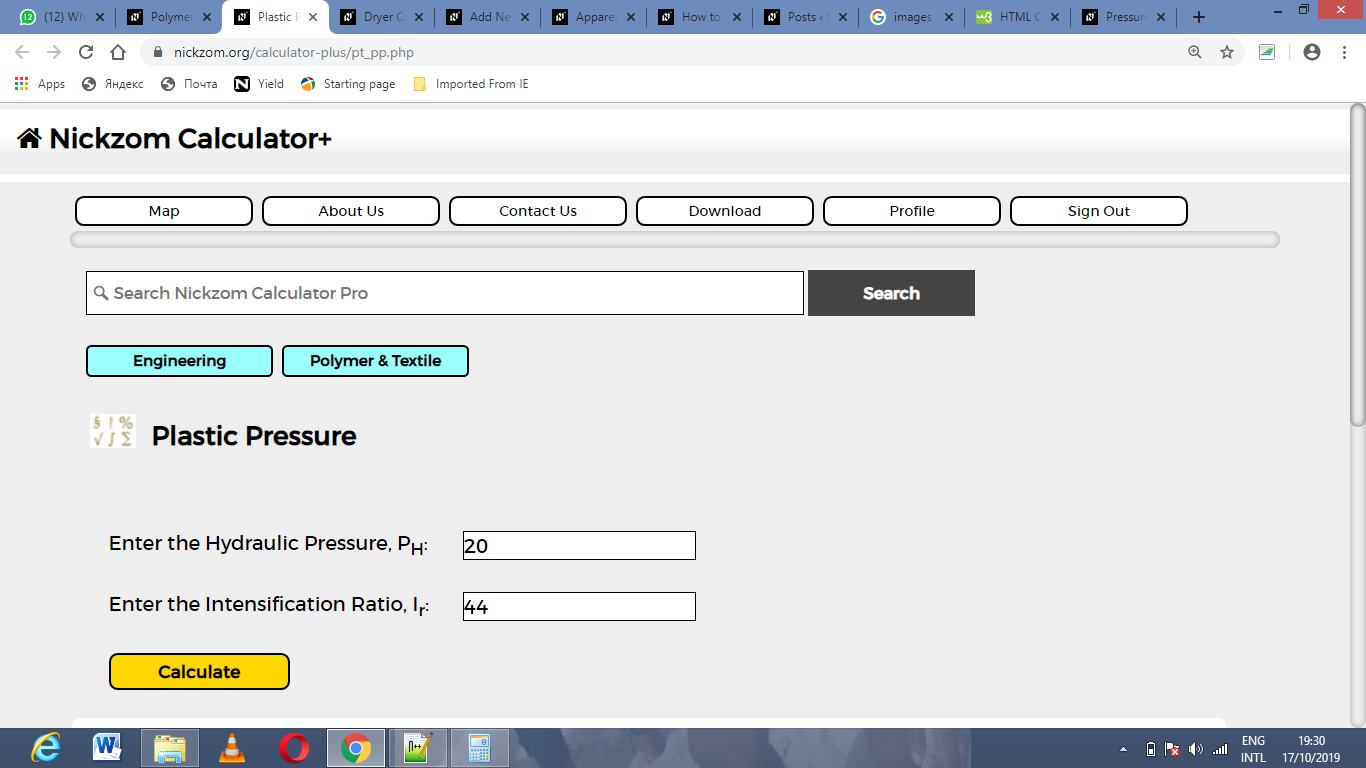Finally, Click on Calculate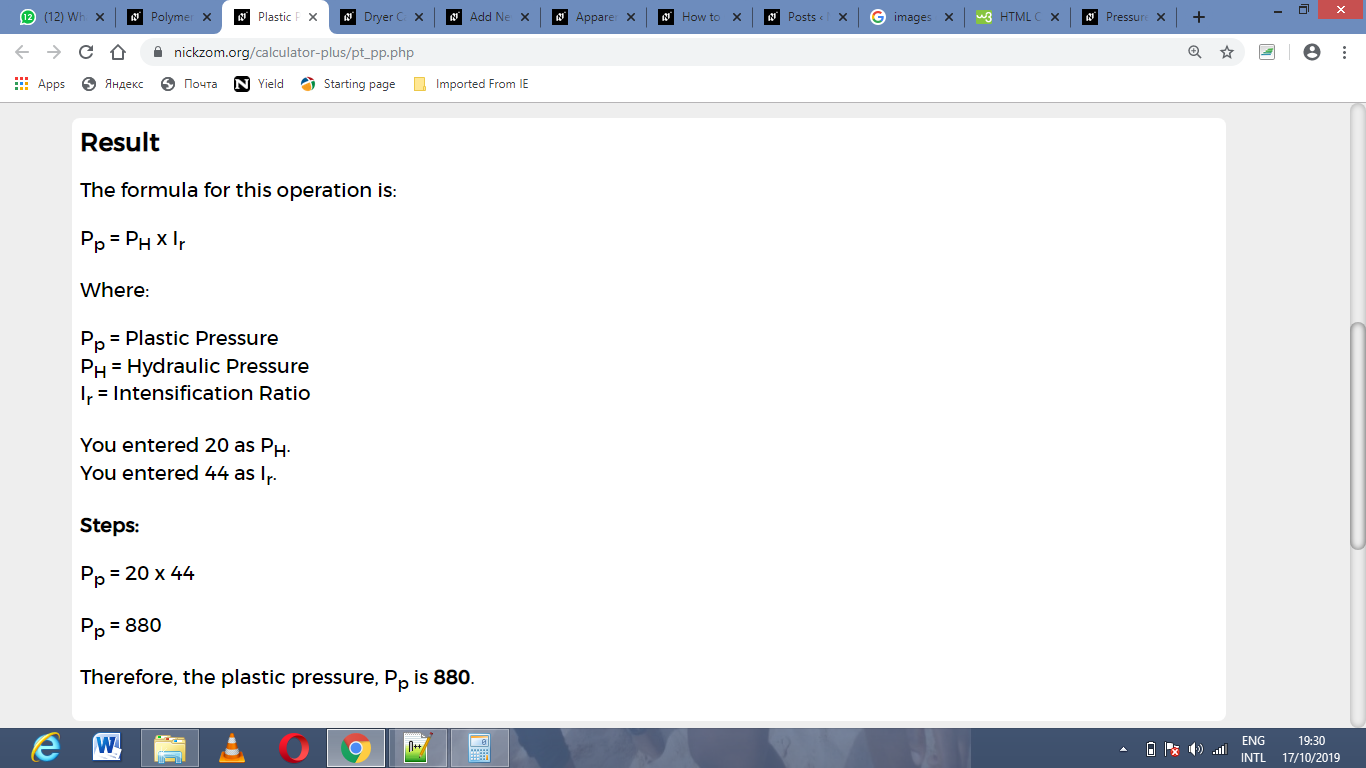As you can see from the screenshot above, Nickzom Calculator– The Calculator Encyclopedia solves for the plastic pressure and presents the formula, workings and steps too.